Physics - 2014 Past Year Paper

# Physics - 2014 Past Year Paper - IIT JAM

Test Description

## 35 Questions MCQ Test IIT JAM Past Year Papers and Model Test Paper (All Branches) - Physics - 2014 Past Year Paper

Physics - 2014 Past Year Paper for IIT JAM 2023 is part of IIT JAM Past Year Papers and Model Test Paper (All Branches) preparation. The Physics - 2014 Past Year Paper questions and answers have been prepared according to the IIT JAM exam syllabus.The Physics - 2014 Past Year Paper MCQs are made for IIT JAM 2023 Exam. Find important definitions, questions, notes, meanings, examples, exercises, MCQs and online tests for Physics - 2014 Past Year Paper below.
Solutions of Physics - 2014 Past Year Paper questions in English are available as part of our IIT JAM Past Year Papers and Model Test Paper (All Branches) for IIT JAM & Physics - 2014 Past Year Paper solutions in Hindi for IIT JAM Past Year Papers and Model Test Paper (All Branches) course. Download more important topics, notes, lectures and mock test series for IIT JAM Exam by signing up for free. Attempt Physics - 2014 Past Year Paper | 35 questions in 90 minutes | Mock test for IIT JAM preparation | Free important questions MCQ to study IIT JAM Past Year Papers and Model Test Paper (All Branches) for IIT JAM Exam | Download free PDF with solutions
 1 Crore+ students have signed up on EduRev. Have you?
Physics - 2014 Past Year Paper - Question 1

### For vectors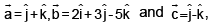the vector product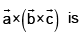Physics - 2014 Past Year Paper - Question 2

### A particle of mass m carrying charge q is moving in a circle in a magnetic field B. According to the Bohr’s model, the energy of the particle in the nth level is _____.

Physics - 2014 Past Year Paper - Question 3

### A conducting slab of copper PQRS is kept on the xy plane in a uniform magnetic field along xaxis as indicated in the figure. A steady current I flows through the cross- section of the slab along the y- axis. The direction of the electric field inside the slab, arising due to the applied magnetic field is along the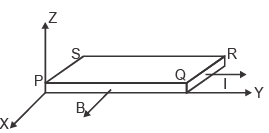Physics - 2014 Past Year Paper - Question 4

A collimated beam of light of diameter 1 mm is propagating along the x- axis. The beam is to be expanded 10 mm using a combination of two convex lenses. A lens of focal length of 50 mm and another lens with focal length F are to be kept at a distance d between them. The values of F and d respectively, are

Physics - 2014 Past Year Paper - Question 5

Octal equivalent of decimal number 47810 is

Physics - 2014 Past Year Paper - Question 6

A spherical ball of ice has radius R0 and is rotating with an angular speed w about an axis passing through its centre. At time t = 0, it starts acquiring mass because the moisture around it starts to freeze on it uniformly. As a result its radius increases as R(t) = R0 + αt, where a is a constant.
The curve which best describes its angular speed with time is

Physics - 2014 Past Year Paper - Question 7

In a photoelectric effect experiment, ultraviolet light of wavelength 320 nm falls on the photocathode with work function of 2.1 eV. The stopping potential should be close to

Physics - 2014 Past Year Paper - Question 8

In 1- dimension, an ensemble of N classical particles has energy of the form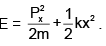The average internal energy of the system at temperature T is ___ .

Physics - 2014 Past Year Paper - Question 9

In an ideal operational amplifier depicted below, the potential at node A is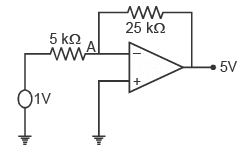Physics - 2014 Past Year Paper - Question 10

To operate a npn transistor in active region, its emitter- base and collector- base junction respectively, should be

Physics - 2014 Past Year Paper - Question 11

The value of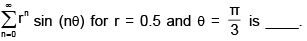Physics - 2014 Past Year Paper - Question 12

In a parallel plate capacitor the distance between the plates is 10 cm. Two dielectric slabs of thick- ness 5 cm each and dielectric constants K1= 2 and K2 = 4 respectively, are inserted between the plates. A potential of 100 V is applied across the capacitor as shown in the figure. The value of the net bound surface charge density at the interface of the two dielectrics is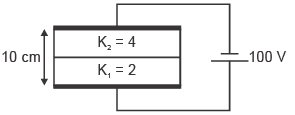Physics - 2014 Past Year Paper - Question 13

The electric fields of two light sources with nearby frequencies ω1 and ω2, and wave vectors k1 and k2, are expressed as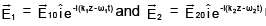respectively. The interference pattern on the screen is photographed at t = t0; denote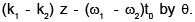For this pattern

Physics - 2014 Past Year Paper - Question 14

A solid metallic cube of heat capacity S is at temperature 300 K. It is brought in contact with a reservoir at 600 K. If the heat transfer takes place only between the reservoir and the cube, the entropy change of the reservoir after reaching the thermal equilibrium is ____

Physics - 2014 Past Year Paper - Question 15

If the surface integral of the field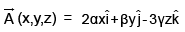over the closed surface of an arbitrary unit sphere is to be zero, then the relationship between α, β  and γ is

Physics - 2014 Past Year Paper - Question 16

The moment of inertia of a disc about one of its diameters is IM. The mass per unit area of the disc is proportional to the distance from its centre. If the radius of the disc is R and its mass is M, the value of IM is _______

Physics - 2014 Past Year Paper - Question 17

The rigid uniform horizontal wire PQ of mass M, pivoted at P, carries a constant current I. It rotates with a constant angular speed in a uniform vertical magnetic field B. If the current were switched off, the angular acceleration of the wire, in terms of B, M and I would be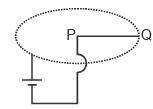Physics - 2014 Past Year Paper - Question 18

Two points N and S are located in the northern and southern hemisphere, respectively, on the same longitude. Projectiles P and Q are fired from N and S, respectively, towards each other.Which of the following option is correct for the projectiles as they approach the equator ?

Physics - 2014 Past Year Paper - Question 19

Two particles A and B of mass m and one particle C of mass are kept on the x axis in thr order ABC, Particle A is given a velocity vi . Consequently there are two collisions, both of which are completely inelastic. If the net energy loss because of these collisions is 7/8 of the initial energy,, the value of M is (ignore frictional losses)

Physics - 2014 Past Year Paper - Question 20

The line integral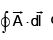of a vector field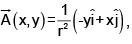where r2 = x2 + y2, is taken around a square (see figure) of side unit length and centered at (x0, y0) with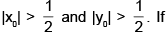the value of the integral is L, then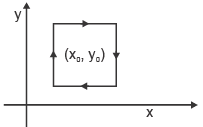Physics - 2014 Past Year Paper - Question 21

Diamond lattice can be considered as a combination of two fcc lattices displaced along the body diagonal by one quarter of its length. There are eight atoms per unit cell. The packing fraction of the diamond structure is

Physics - 2014 Past Year Paper - Question 22

Thermal neutrons (energy = 300 kB = 0.025 eV) are sometimes used for structural determination of materials. The typical lattice spacing of a material for which these can be used is

Physics - 2014 Past Year Paper - Question 23

What is the maximum height above the dashed line attained by the water stream coming out at B from a thin tube of the water tank assembly shown in the figure? Assume h = 10 m, L = 2m, and θ = 30o.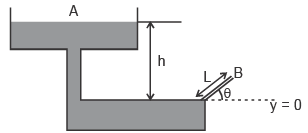Physics - 2014 Past Year Paper - Question 24

A steady current in a straight conducting wire produces a surface charge on it. Let Eout and Ein be the magnitudes of the electric fields just outside and just inside the wire, respectively. W hich of the following statements is true for these fields ?

Physics - 2014 Past Year Paper - Question 25

A small charged spherical shell of radius 0.01 m is at a potential of 30 V. The electrostatic energy of the shell is

Physics - 2014 Past Year Paper - Question 26

At an instant shown, three point masses m, 2m and 3m rest on a horizontal surface, and are at the vertices of an equilateral triangle of unit side length. Assuming that G is the gravitational constant, the magnitude and direction of the torque on the mass 3m, about the point O, at that instant is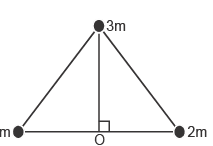Physics - 2014 Past Year Paper - Question 27

A sine wave of 5V amplitude is applied at the input of the circuit shown in the figure. which of the following waveforms represents the output most closely?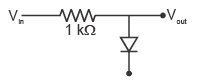Physics - 2014 Past Year Paper - Question 28

1011 binary have been applied at X3 X2 X1 X0 input in the shown logic circuit made of XOR gates. The binary output Y3 Y2 Y1 Y0 of the circuit will be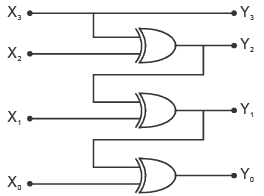Physics - 2014 Past Year Paper - Question 29

A ring of radius R carries a linear charge density l.  It is rotating with angular speed w.  The magnetic field at its center is

Physics - 2014 Past Year Paper - Question 30

A stationary source (see figure below) emits sound waves of frequency f towards a wall. If an observer moving with speed u in a direction perpendicular to the wall, measures a frequency f’ = 9/8 f at the instant shown, then u is related to the speed of sound Vs as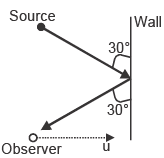Physics - 2014 Past Year Paper - Question 31

A real gas has specific volume v at temperature T. Its coefficient of volume expansion and isothermal compressibility are a and kT, respectively. Its molar specific heat at constant pressure Cp and molar specific heat at constant volume Cv are related as

Physics - 2014 Past Year Paper - Question 32

Two frames, O and O’, are in relative motion as shown. O’ is moving with speed c/2, where c is the speed of light. In frame O, two separate events occur at (x1, t1) and (x2, t2). In frame O’, these events occur simultaneously. The value of (x2 - x1)(t2 - t1) is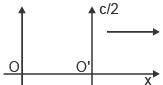Physics - 2014 Past Year Paper - Question 33

White light is incident on a grating G1 with groove density 600 lines/mm and width 50 mm. A small portion of the diffracted light is incident on another grating G2 with groove density 1800 lines/mm and width 15 mm. The resolving power of the combined system is

Physics - 2014 Past Year Paper - Question 34

Four particles of mass m each are inside a two dimensional square box of side L. If each state obtained from the solution of the Schrodinger equation is occupied by only one particle, the minimum energy of the system in units of  h2/mL2 is

Physics - 2014 Past Year Paper - Question 35

At atmospheric pressure (= 105 Pa), aluminium melts at 550 K. As it melts, its density decreases from 3 x 103 kg/m3 to 2.9 x 103 kg/m3. Latent heat of fusion of aluminium is 24 x 103 J/kg. The melting point of aluminium at a pressure of 107 Pa is closest to

## IIT JAM Past Year Papers and Model Test Paper (All Branches)

8 docs|48 tests
Information about Physics - 2014 Past Year Paper Page
In this test you can find the Exam questions for Physics - 2014 Past Year Paper solved & explained in the simplest way possible. Besides giving Questions and answers for Physics - 2014 Past Year Paper, EduRev gives you an ample number of Online tests for practice

## IIT JAM Past Year Papers and Model Test Paper (All Branches)

8 docs|48 tests(Scan QR code)